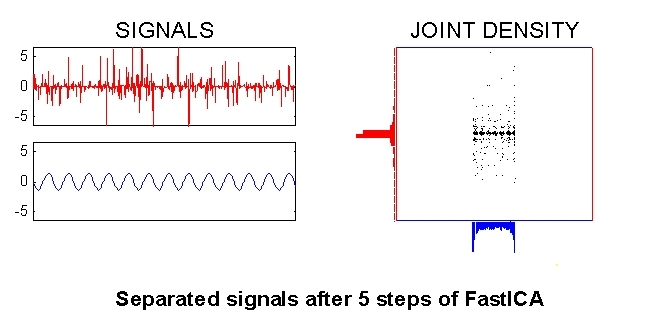# What is Independent Component Analysis: A Demo

Independent Component Analysis (ICA) is a statistical technique for decomposing a complex dataset into independent sub-parts. Here, we demonstrate ICA for solving the Blind Source Separation (BSS) problem.

We are given two linear mixtures of two source signals which we know to be independent of each other, i.e. observing the value of one signal does not give any information about the value of the other. The BSS problem is then to determine the source signals given only the mixtures.

Putting this into mathematical notation, we model the problem by

`x = As`
where s is a two-dimensional random vector containing the independent source signals, A is the two-by-two mixing matrix, and x contains the observed (mixed) signals.

This first plot (below) shows the signal mixtures on the left and the corresponding joint density plot on the right. That is, at a given time instant, the value of the top signal is the first component of x, and the value of the bottom signal is the corresponding second component. The plot on the right is then simply constructed by plotting each such point x. The marginal densities are also shown at the edge of the plot.

#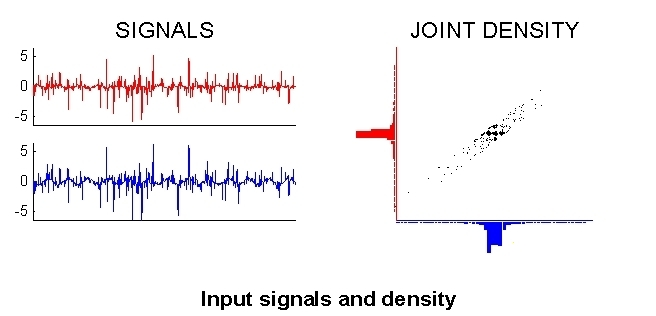A first step in many ICA algorithms is to whiten (sphere) the data. This means that we remove any correlations in the data, i.e. the signals are forced to be uncorrelated. Again putting the words in mathematical terms, we seek a linear transformation V such that when y = Vx we now have E{yy'} = I. This is easily accomplished by setting V = C-1/2, where C = E{xx'} is the correlation matrix of the data, since then we have E{yy'} = E{Vxx'V'} = C-1/2CC-1/2 = I.

The figure below shows the signals y and the joint density p(y) after such an operation.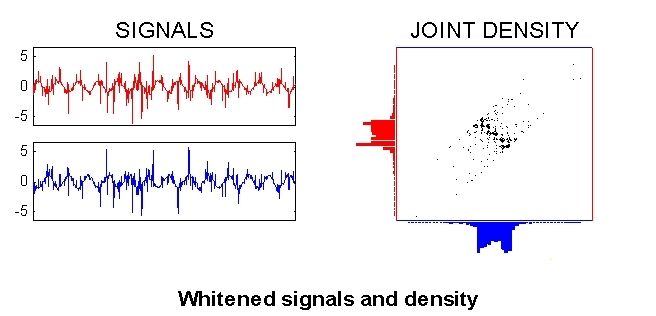After sphering, the separated signals can be found by an orthogonal transformation of the whitened signals y (this is simply a rotation of the joint density). The appropriate rotation is sought by maximizing the non-normality of the marginal densities (shown on the edges of the density plot). This is because of the fact that a linear mixture of independent random variables is necessarily more Gaussian than the original variables. (This is the same phenomenon as is stated by the central limit theorem.) This implies that in ICA we must restrict ourselves to at most one Gaussian source signal.

There are many algorithms for performing ICA, but the most efficient to date is the FastICA. (fixed-point) algorithm which was developed by us. The plot below shows the result after one step of the FastICA algorithm.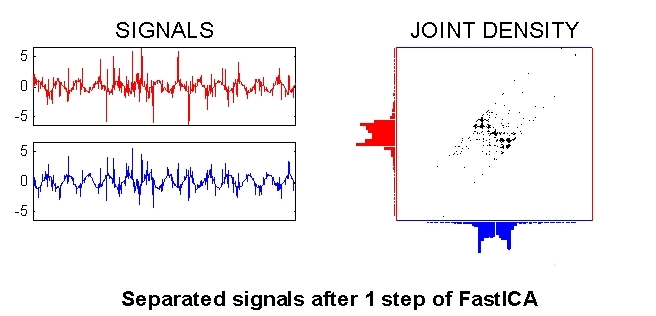The rotation continues...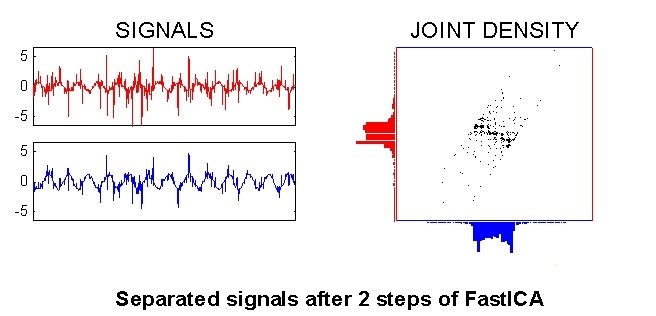...and continues......until it starts to converge...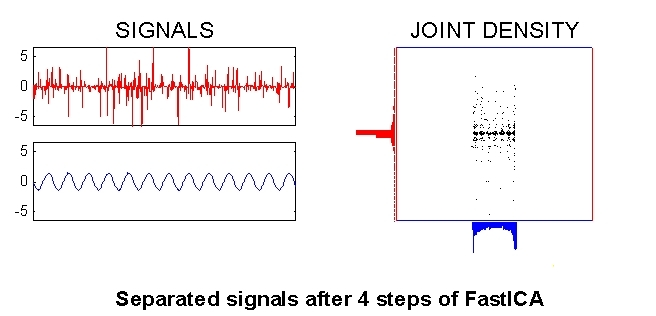Convergence! The source signals (components of s) in this example were a sinusoid and impulsive noise, as can be seen in the left part of the plot below. The right plot shows the joint density which can be seen to be the product of the marginal densities, i.e. p(s)=p(s1)p(s2). This is of course the definition of independence.